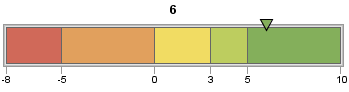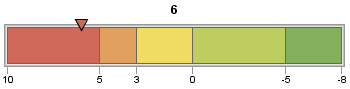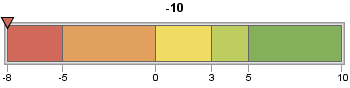# Specifying Qualitative Range Boundaries and Actual KPI Values

To generate a KPI chart, you must specify a list of range boundaries using the BOUNDS= option and an actual KPI value using the ACTUAL= option. The values can be positive numbers, negative numbers, or missing (ACTUAL=.), but the BOUNDS= list must be in either ascending or descending order and must contain at least two numbers (in order to define a single range). For example, the following code defines a horizontal slider with range boundaries in ascending order from –8 to 10 and an actual KPI value of 6:
```goptions device=javaimg;
proc gkpi;
hslider actual=6 bounds=(-8 -5 0 3 5 10);
run;
quit;```The boundaries can also be specified in desending order, for example:
`hslider actual=6 bounds=(10 5 3 0 -5 -8)`The order in which colors are applied is not affected by whether boundaries are specified in ascending or descending order. See Defining Active and Inactive Color Lists for information about controlling range colors.
The actual KPI value can fall outside of the highest or lowest boundaries, but the GKPI procedure treats such values as if they occur at the edge of the highest or lowest boundaries. For example, suppose the actual KPI value is –10, but the lowest boundary value is –8:
`hslider actual=-10 bounds=(-8 -5 0 3 5 10)`
PROC GKPI displays the actual KPI value indicator at –8.If you specify a missing value for the actual KPI value (ACTUAL=.), then the GKPI procedure does not generate a KPI chart.